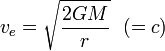The Schwarzschild radius is that distance from the center of gravity of a massive body from where the escape speed is equal to the speed of light. If the radius of the object itself is smaller than its Schwarzschild radius, it will not let light escape and will thus be a black hole. In the case of the blackhole, the Schwarzschild radius is effectively its event horizon, the perceived limit of the black hole. Pertaining to black holes, event horizon is an equivalent term.

##  Physics

The escape speed is a concept of mechanics which is related to gravity. For a small body being launched radially outwards at a radius r from a larger body of mass M, the escape speed ve is that speed which would cause the small object to come at rest at a radius of infinity from the large object (where the force of gravity is exactly equal to zero). In mathematical form, following from the definition of gravitational potential, this can be approximated as:$v_e = \sqrt{\frac{2GM}{r}}\ \ (= c)$

where G is the gravitational constant. The Schwarzschild radius is the special case such that ve = c (the speed of light). The above formula can be rearranged more usefully into:$r_{Schwarzschild} = \frac{2GM}{c^2}$

Please note that this formula relies on the assumption that the Schwarzschild radius is much larger than the radius of the body in question, and thus serves more as an illustration than an actual definition.Some content on this page may previously have appeared on Citizendium.